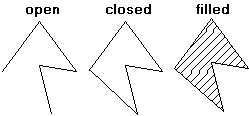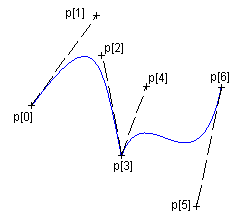## Open, Closed and Filled Polygons, Bezier Lines and Regions Creation

The functions cdBegin, cdVertex and cdEnd are use for many situations. cdBegin is called once, cdVertex can be called many times, and cdEnd is called once to actually do something. If you call cdBegin again before cdEnd the process is restarted, except for cdBegin(CD_REGION) that can contains one or more polygons inside.

```void cdCanvasBegin(cdCanvas* canvas, int mode); [in C]

canvas:Begin(mode: number) [in Lua]```

Starts defining a polygon to be drawn (or filled) according to the mode:  CD_CLOSED_LINES, CD_OPEN_LINES, CD_FILL, CD_CLIP, CD_REGION or CD_BEZIER. Do not create embedded polygons, that is, do not call function cdBegin twice without a call to cdEnd in between.

• CD_OPEN_LINES: connects all the points at cdEnd. Depends on line width and line style attributes.
• CD_CLOSED_LINES: connects all the points at cdEnd and connects the last point to the first.  Depends on line width and line style attributes.
• CD_FILL: connects the last point to the first and fills the resulting polygon according to the current interior style. When the interior style CD_HOLLOW is defined the it behaves as if the mode were CD_CLOSED_LINES.
• CD_CLIP: instead of creating a polygon to be drawn, creates a polygon to define a polygonal clipping region.
• CD_BEZIER: defines the points of a bezier curve. There must be at least 4 points: start, control, control and end. To specify a sequence of curves use 3 more points for each curve: control, control, end, control, control, end, ... The end point is used as start point for the next curve.
• CD_REGION: starts the creation of a complex region for clipping. All calls to cdBox, cdSector, cdChord, Filled Polygons and cdText will be composed in a region for clipping. See Regions documentation.
• CD_PATH: creates a path composed of several primitives that can be line draw, filled or used as clipping. Must call cdCanvasPathSet to configure the action between sequences of cdCanvasVertex.

Open, Closed and Filled PolygonsBezier Lines```void cdCanvasVertex(cdCanvas* canvas, int x, int y); [in C]
void cdfCanvasVertex(cdCanvas* canvas, double x, double y); [in C]
void wdCanvasVertex(cdCanvas* canvas, double x, double y); (WC) [in C]

canvas:Vertex(x, y: number) [in Lua]
canvas:wVertex(x, y: number) (WC) [in Lua]```

Adds a vertex to the polygon definition.

```void cdCanvasEnd(cdCanvas* canvas); [in C]

canvas:End() [in Lua]```

Ends the polygon's definition and draws it.

```void cdCanvasPathSet(cdCanvas* canvas, int action); [in C]

canvas:PathSet(action: number) [in Lua]```

Configures the action between sequences of cdCanvasVertex. action can be:

•  CD_PATH_NEW - creates a new empty path. Useful if more than one path is configured. cdCanvasBegin(CD_PATH) already creates a new path.
• CD_PATH_MOVETO - moves the current position to the given coordinates. Must be followed by 1 call to cdCanvasVertex, cdfCanvasVertex, or wdCanvasVertex.
• CD_PATH_LINETO - adds a line to the path from the current position to the given coordinates. The current position is updated to the  given coordinates. If there is no current position, nothing is connected and only the current position is updated. Must be followed by 1 call to cdCanvasVertex, cdfCanvasVertex, or wdCanvasVertex.
• CD_PATH_ARC - adds an arc to the path. If there is a current position adds also a line from the current position to the start of the arc. The end of the arc becomes the current position. Must be followed by 3 calls to cdCanvasVertex, cdfCanvasVertex, or wdCanvasVertex. One for the center of the arc (xc,yc), one for the bounding rectangle size (w,h), and one for the start and end angles (angle1,angle2). Angles are in degrees and oriented counter-clockwise, but angle2 can be smaller than angle1 to describe a clockwise arc. When using integer coordinates angles must be multiplied by 1000.
• CD_PATH_CURVETO - adds a bezier curve to the path. If there is no current position, the first point will be used twice. The end point becomes the current position. Must be followed by 3 calls to cdCanvasVertex, cdfCanvasVertex, or wdCanvasVertex. Must be first control point (x1,y1) + second control point (x2,y2) + end point (x3,y3).
• CD_PATH_CLOSE - adds a line to the path that connects the last point with the first point of the path, closing it.
• CD_PATH_FILL - fills the path with the current fill attributes, then the path is discarded.
• CD_PATH_STROKE - strokes the path with the current line attributes, then the path is discarded.
• CD_PATH_FILLSTROKE - fills the path with the current fill attributes, strokes the path with the current line attributes, then the path is discarded.
• CD_PATH_CLIP - use the path as a clipping area to be intersected with the current clipping area, then the path is discarded. This will also set the current clip mode to CD_CLIPPATH (since 5.12)

So the normal path creation to draw a line will do:

```cdCanvasBegin(canvas, CD_PATH);
cdCanvasPathSet(canvas, CD_PATH_MOVETO);
cdCanvasVertex(canvas, x1, y1);
cdCanvasPathSet(canvas, CD_PATH_LINETO);
cdCanvasVertex(canvas, x2, y2);
cdCanvasPathSet(canvas, CD_PATH_CURVETO);
cdCanvasVertex(canvas, x3, y3);  /* control point for start point */
cdCanvasVertex(canvas, x4, y4);  /* control point for end point */
cdCanvasVertex(canvas, x5, y5);  /* end point */
cdCanvasPathSet(canvas, CD_PATH_ARC);
cdCanvasVertex(canvas, x6, y6);  /* center */
cdCanvasVertex(canvas, x7, y7);  /* width, height */
cdCanvasVertex(canvas, x8, y8);  /* start angle, end angle (degrees / 1000) */
cdCanvasPathSet(canvas, CD_PATH_STROKE);
cdCanvasEnd(canvas);```